ElectroMagnetic induction

(6) Motional EMF

• We know that an emf is produced in the loop when the amount of magnetic flux linked with the circuit os changed.
• The flux Φ linked with the loop can be changed by
(i) Keeping the loop at rest and changing the magnetic field i.e , there is no physical movemnt of either the source of emf or the loop(or coil) through which the magnetic flux is linked but the magnetic field changes with time and this may caused by changing the electric current producing the field
(ii)Keeping the magnetic field constant and moving the loop or source of the magnetic field partly or wholey i.e the change is produced by the relative motion of the source of the magnetic field and the lopp (or coil) through which the magnetic field passes.
• In both the cases of producing the emf,the induced emf is given by the same law i.e it is equal to the time rate of change of the magnetic flux.
• In the later case emf induced due to the relative motion of source of magnetic field and coil is called motional emf.
• This phenomemon of motionla emf can be understood easily in lorentz force on moving charges
• Consider a thin conducting rod AB length l moving in the magnetic field B with constant velocity v as shown in the below in the figure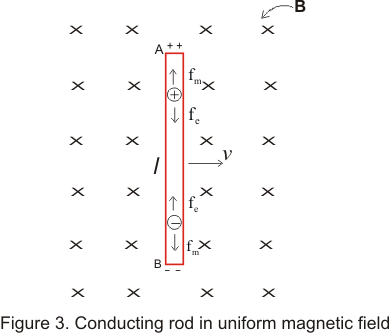• This uniform magnetic field B is perpendicular to the plane of the diagram ,directed away from the reader
• Rod is moving in the magnetic field in such a way that its velocity is perpendicular to the magnetic field B and its own axis
• when we move conducting rod AB with velocity v,free electrons in it gain velocity in the direction of the motion and in the presence of magnetic field ,these free electrons experiences lorentz force perpendicular to both B and v
• The electrons under this force accumulate at end end ,providing it negative polarity and the other end deprived of the electrons becomes positively charged
• Magnetic force or lorentz force Fm acting on these moving electrons is Fm=qv X B
where q=-1.6*10-19 C ,charge on each electron
• According to fleming left hand rule force on negative charges is towards B hence negative charge accumulates at B and positive charge appears at A
• As charges accumulates at the ends of the rod,an electric field E is produced in the rod from A to B.This field E is of non electrostatics origins and is produced by the changing magnetic fields
• This electric field in turns produces a force on electron in the conducting rod which is opposite to the lorentz force so
Fe=qE
• when enough charge accumulates a situation comes when this electric force cancels out lorentz force and then free electrons do not drift anymore.In this situation
Fm =Fe or |qv X B |=|qE| or vB=E
• In this situation there is no force acting on the free electrons of the rod AB. Potential difference between the ends A and B of the rod would be
V=El=vBl This is the emf induced in the rod AB due to its motion in the magnetic field
• Thus motional emf induced in the rod moving in magnetic field is ξ=vBl ----(6)
• If the velocity v of the rod makes an angle θ with the direction of the magnetic field ,the potential difference induced between the ends of the conductor will be
ξ=vBlsinθ
as vsinθ is the component of v perpendicular to B
• If the rod moves parallel to the field i.e, θ =0 no potential difference will be induced
• The emf associated with the moving rod in Figure 3 is analogous to that of a battery with the positive terminal at A and negative terminal at B
• If ends of A and B are connected by an external resistor and if r is the internal resistance of the rod then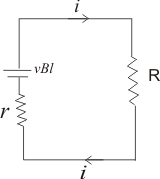an electric field is produced in this resitor due to the potential differebce and a current is established in the circuit with direction from A to B in the external circuit
• Since magnitude of current of induced emf is
ξ=vBl
So current is,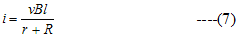and direction of current can be found using lenz's law
• We now know the current flowing in the circuit from this we can calculate the power loss and force F connected with this motion Thus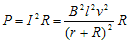if r <<< R then it can be neglected so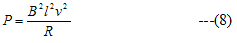and Force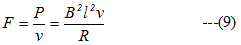Note to our visitors :-

Thanks for visiting our website.
DISCLOSURE: THIS PAGE MAY CONTAIN AFFILIATE LINKS, MEANING I GET A COMMISSION IF YOU DECIDE TO MAKE A PURCHASE THROUGH MY LINKS, AT NO COST TO YOU. PLEASE READ MY DISCLOSURE FOR MORE INFO.